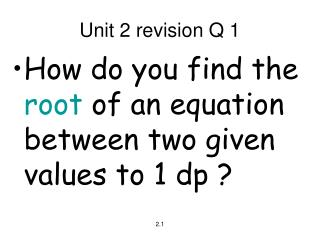# Unit 2 revision Q 1 - PowerPoint PPT PresentationDownload PresentationUnit 2 revision Q 1

Unit 2 revision Q 1
Download Presentation## Unit 2 revision Q 1

- - - - - - - - - - - - - - - - - - - - - - - - - - - E N D - - - - - - - - - - - - - - - - - - - - - - - - - - -
##### Presentation Transcript

1. Unit 2 revision Q 1 • How do you find the root of an equation between two given values to 1 dp ? 2.1

2. Answer to Unit 2 revision Q 1 • iteration

3. Unit 2 revision Q 2 • How do you find where a curve crosses the x-axis ? 2.1

4. Answer to Unit 2 revision Q 2 • Let y = 0 or f(x) = 0 and solve for x

5. Unit 2 revision Q 3 • How do you solve equations like 100 x2 4 - =0? 2.1

6. Answer to Unit 2 revision Q 3 • (i) multiply by the denominator of the fraction (here x2) • (ii) factorise and solve

7. Unit 2 revision Q 4 • What is the condition for equal roots ? 2.1

8. Answer to Unit 2 revision Q 4 • b2 – 4ac = 0

9. Unit 2 revision Q 5 • What is the quadratic formula and explain when it is used ? 2.1

10. Answer to Unit 2 revision Q 5 • x = -b±√(b2-4ac) 2a • It is used to find roots of a quadratic equation when it is difficult to factorise.

11. Unit 2 revision Q 6 • How do you solve quadratic inequations like x2+5x-6 ≥ 0 ? 2.1

12. Answer to Unit 2 revision Q 6 • (i) factorise • (ii) draw graph • (iii) read x values where graph is on or above x-axis

13. Unit 2 revision Q 7 • How do you solve quadratic inequations like x2 - 5x + 6 < 0 ? 2.1

14. Answer to Unit 2 revision Q 7 • (i) factorise • (ii) draw graph • (iii) read x values where graph is below x-axis

15. Unit 2 revision Q 8 • What is the condition for no real roots ? 2.1

16. Answer to Unit 2 revision Q 8 • b2 – 4ac < 0

17. Unit 2 revision Q 9 • How do you find where a curve crosses the y-axis ? 2.1

18. Answer to Unit 2 revision Q 9 • Substitute x=0 into equation to find y value

19. Unit 2 revision Q 10 • What is the condition for real roots ? 2.1

20. Answer to Unit 2 revision Q 10 • b2 – 4ac ≥ 0

21. Unit 2 revision Q 11 • How do you find where a line and a circle intersect ? 2.4

22. Answer to Unit 2 revision Q 11 • Rearrange line to get x = … or y = … • Substitute into circle equation and solve

23. Unit 2 revision Q 12 • How do you factorise a cubic expression like x3-2x2-x+2 ? 2.1

24. factor 1 -2 -1 2 Remainder=0 Answer to Unit 2 revision Q 12 • Synthetic division using factors of last number

25. Unit 2 revision Q 13 • How do you find ∫ x3 dx ? b a 2.2

26. Answer to Unit 2 revision Q 13 [ ] • x3+1 3+1 then 1/4[(b4) - (a4)] b a

27. Unit 2 revision Q 14 • How do you integrate xn ? 2.2

28. Answer to Unit 2 revision Q 14 • xn+1 n+1 + C

29. Unit 2 revision Q 15 • How do you calculate the area under a curve ? 2.2

30. Answer to Unit 2 revision Q 15 • (i) integrate • (ii) substitute in two limits and subtract to find area

31. Unit 2 revision Q 16 • How do you find the exact values of sin(A+B), cos(A-B) etc. given that • cosA = 3/5 and • sinB = 12/13 ? 2.3

32. A 5 3 13 12 Answer to Unit 2 revision Q 16 • (i) draw two Δs • (ii) find missing sides • (iii) expand formula • (iv) substitute in values from Δs using SOH CAH TOA B

33. Unit 2 revision Q 17 • How do you solve equations like • Cos2xo - 5cosxo = 2 ? (0 ≤ x ≤ 360) 2.3

34. Answer to Unit 2 revision Q 17 • (i) Substitute 2cos2xo-1 for cos2xo • (ii) Make RHS=0 and then factorise (use c for cos xo if you like) • (iii) solve for cos xo and hence for x

35. Unit 2 revision Q 18 • What is the centre and radius of a circle of the form • x2+y2+2gx+2fy+c=0 ? 2.4

36. Answer to Unit 2 revision Q 18 • Centre (-g,-f) • Radius √(g2+f2-c)

37. Unit 2 revision Q 19 • What is the centre and radius of a circle with equation x2 + y2 = r2 ? 2.4

38. Answer to Unit 2 revision Q 19 • (i) centre (0,0) • (ii) radius = r

39. Unit 2 revision Q 20 • What is the centre and radius of a circle of the form • (x-a)2+(y-b)2 = r2 ? 2.4

40. y r C (a,b) x Answer to Unit 2 revision Q 20 • Centre (a,b) • Radius = r